# 基于C#+asp.net+C++的RSA文件加密系统设计与实现（毕业论文+程序源码）

## 1、项目简介

1. 本文设计的是一套完整实用的RSA文件加密解决方案，并具体编码实现。本文采用费马小定理测试素数，使用Montgomery加快大数模乘运算，用C++实现RSA加密算法类库，并在32位windows平台封装成组件。在.Net平台引用此组件，实现可以对任意文件进行RSA加密操作的窗体应用程序。经过加密的文件以及密钥文件都是文本文件。本文首先给出关键类类图、整个应用程序的结构描述文档，然后对关键模块流程图、详细的接口文档进行阐述，并给出关键的实现代码，最后对应用程序进行测试，对测试结果进行分析研究，进而对应用程序进行改进，对关键算法进行尽可能的优化，最终得到一个在windows运行的可以用指定密钥对任意文件进行RSA加密并可解密的完整应用程序，和一些相关的可移植组件。

## 2、资源详情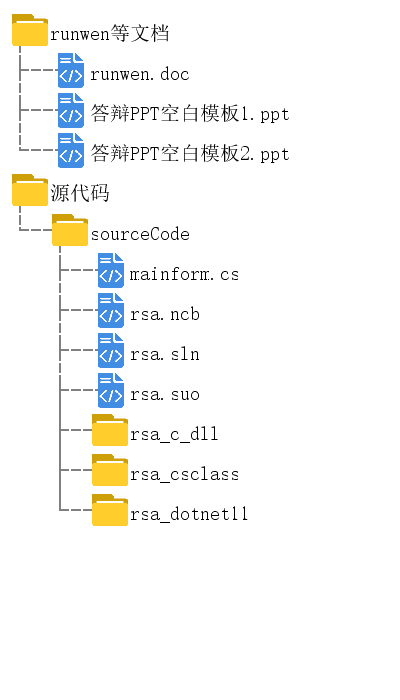## 3、关键词：

RSA；文件加密；Montgomery；费马定理

## 4、毕设简介

1.1课题背景
RSA公钥加密算法是第一个既能用于数据加密也能用于数字签名的算法。它易于理解和操作，也十分流行。算法的名字以发明者的姓氏首字母命名：Ron Rivest, Adi Shamir 和Leonard Adleman。虽然自1978年提出以来，RSA的安全性一直未能得到理论上的证明，但它经历了各种攻击，至今（2006年）未被完全攻破。随着越来越多的商业应用和标准化工作，RSA已经成为最具代表性的公钥加密技术。VISA、MasterCard、IBM、Microsoft等公司协力制定的安全电子交易标准（Secure Electronic Transactions，SET）就采用了标准RSA算法，这使得RSA在我们的生活中几乎无处不在。网上交易加密连接、网上银行身份验证、各种信用卡使用的数字证书、智能移动电话和存储卡的验证功能芯片等，大多数使用RSA技术。

1.2 RSA算法介绍与应用现状
RSA算法可以简单叙述如下：
<密钥生成>

<加密解密>
b=ae mod n，c=bd mod n.

RSA公开密钥加密算法自20世纪70年代提出以来，已经得到了广泛认可和应用。发展至今，电子安全领域的各方面已经形成了较为完备的国际规范。RSA作为最重要的公开密钥算法，在各领域的应用数不胜数。RSA在硬件方面，以技术成熟的IC应用于各种消费类电子产品。

RSA在软件方面的应用，主要集中在Internet上。加密连接、数字签名和数字证书的核心算法广泛使用RSA。日常应用中，有比较著名的工具包Open SSL(SSL，Security Socket Layer,是一个安全传输协议，在Internet上进行数据保护和身份确认。Open SSL是一个开放源代码的实现了SSL及相关加密技术的软件包，由加拿大的Eric Yang等发起编写的。相关详细介绍见http://www.openssl.org/about/ )。Open SSL应用RSA实现签名和密钥交换，已经在各种操作系统得到非常广泛的应用。另外，家喻户晓的IE浏览器，自然也实现了SSL协议，集成了使用RSA技术的加密功能，结合MD5和SHA1，主要用于数字证书和数字签名，对于习惯于使用网上购物和网上银行的用户来说，几乎天天都在使用RSA技术。

1.3 RSA应用于文件加密的分析
1.3.1 文件加密使用RSA的可行性

1.3.2 文件加密使用RSA的意义

2 RSA文件加密软件的设计与实现
2.1 需求分析与总体设计
2.1.1 功能分析

① 可以按要求的位数生成非对称密钥。
② 可以用指定密钥以RSA算法加密任意一个文件，加密生成的数据为纯文本。
③ 可以装载加密过的文件，并用指定的密钥解密还原出原文件。
④ 提示信息完整、操作舒适、图形界面雅观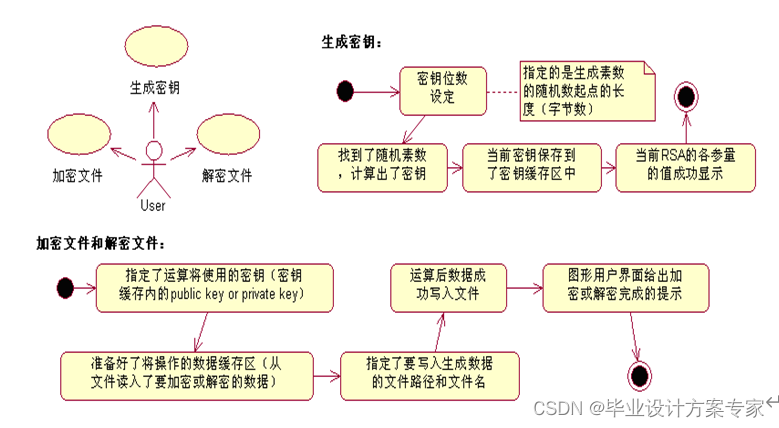①RSA密钥生成 ②RSA加密解密 ③任意文件的读取 ④各环节必要的数据编码转换 ⑤图形操作界面。

2.1.2 工程方案选择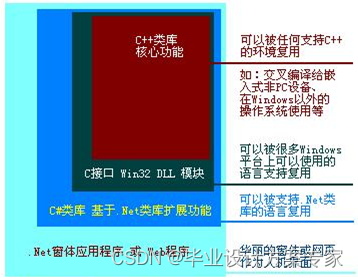2.2 各部分的设计与开发
2.2.1 实现RSA加密算法的C++核心类库
1 大数存储和四则运算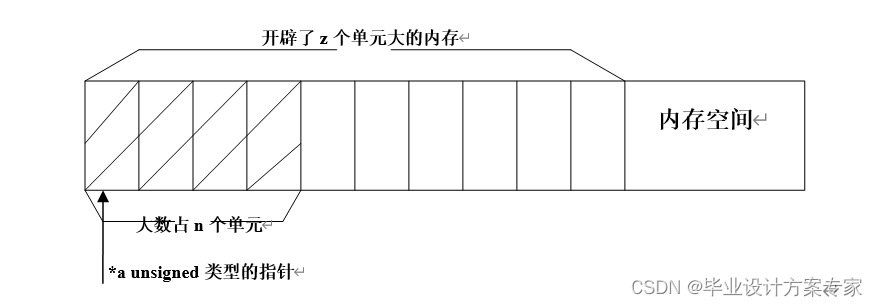2 大数幂模与乘模运算•Montgomery幂模算法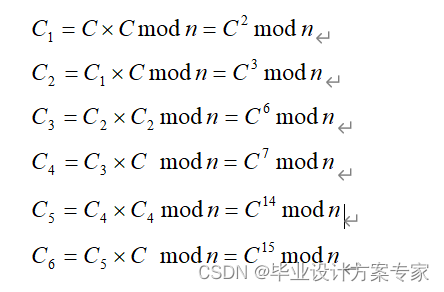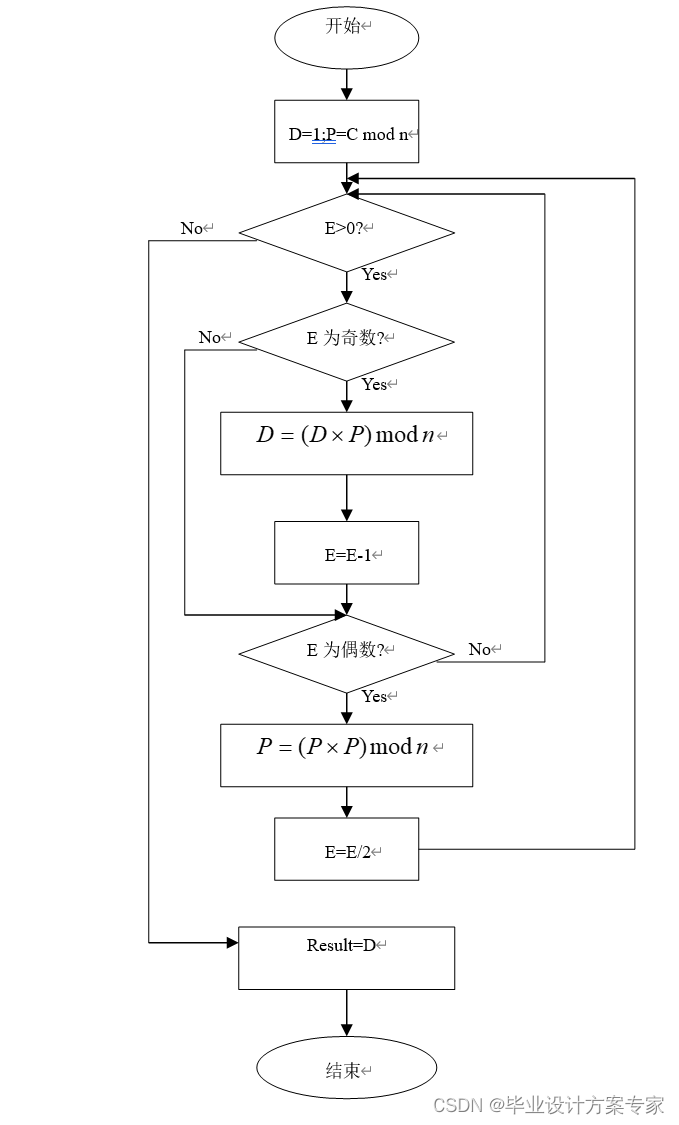① 求 的值

E奇数 D = DP mod n = 2 P = PP mod n = 4 E= (E-1)/2 =7
E奇数 D = DP mod n = 8 P = PP mod n = 16 E= (E-1)/2 =3
E奇数 D = DP mod n = 9 P = PP mod n = 1 E= (E-1)/2 =1
E奇数 D = DP mod n = 9 P = PP mod n = 1 E= (E-1)/2 =0

② 求 的值

E偶数 D = 1 P = PP mod n = 4 E = E/2 =4
E偶数 D = 1 P = PP mod n = 3 E = E/2 =2
E偶数 D = 1 P = PP mod n = 9 E = E/2 =1
E奇数 D = DP mod n = 9 P = 不需要计算 E = (E-1)/2 =0

Powmod(C,E,n)
{
D=1;
P=C mod n;
for i=0 to u do
{
if(Ei=1)D=DP(mod n);
P=P
P(mod n);
}
return D;
}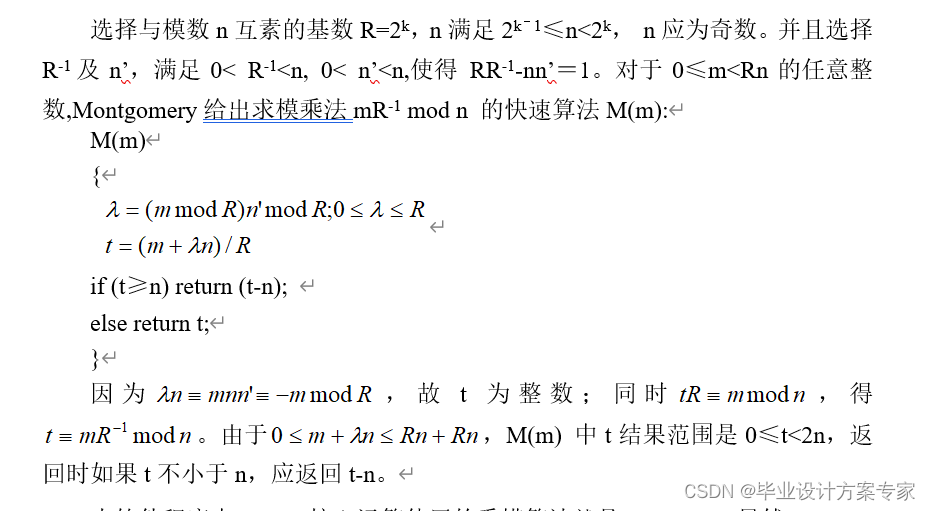M(m) //蒙哥马利乘模

{
k = ( m * n’ ) mod R;
x = (m + k*n ) / R;
if (x>=n) x -= n;
return x;
}
exp(C,E,n) //蒙哥马利幂模

{
D=R-n;
P=CR mod n;
i=0;
while(true)
{
if(E的当前二进制位Ei==1)D=M(D
P); //从低位到高位检测二进制位
i+=1;
if(i==E的二进制位数)break;
P=M(PP);
}
return D
R-1 (mod n);
}

3 寻找素数•Eratosthenes筛选与Fermat素数测试

for (i=0;i<np;i++)
{
unsigned p = pl[i];
unsigned r = start % vlong§;
if ® r = p - r;
while ( r < SS )
{
b[r] = 0;
r += p;
}
}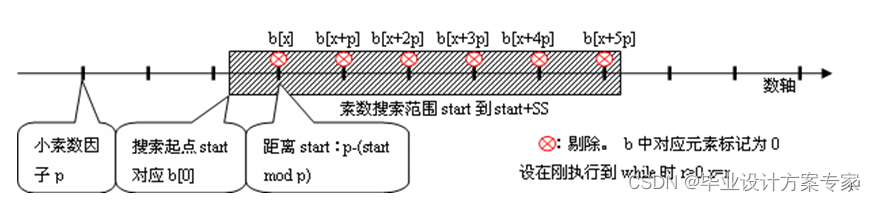int is_probable_prime_san( const vlong &p )
{
const rep = 4; //测试次数
const unsigned any[rep] = { 2,3,5,7 }; //测试用的底数
for ( unsigned i=0; i<rep; i+=1 )
if ( modexp( any[i], p-vlong(1), p ) != vlong(1) ) return 0;
//modexp是幂模函数，按上一小节叙述的算法编码。
//这里modexp计算any[i]p-1mod p。
return 1;
}


(1) 先得到一个随机的大整数N当作寻找的起点．
(2) 确定一个寻找范围的大小SS，把(N,N+SS)范围内的小素数倍数去掉，即前面叙述的古希腊某人发明的筛选法．小素数因子从２开始取，取几百个(论文中将小素数因子个数记为NP)．
(3) 对范围内没有去掉的数逐一进行素数测试，一个数如果通过测试次数达到一定标准，就判为素数．
(4) 如果范围内没找到素数，就令N=N+SS，回到(2)继续寻找．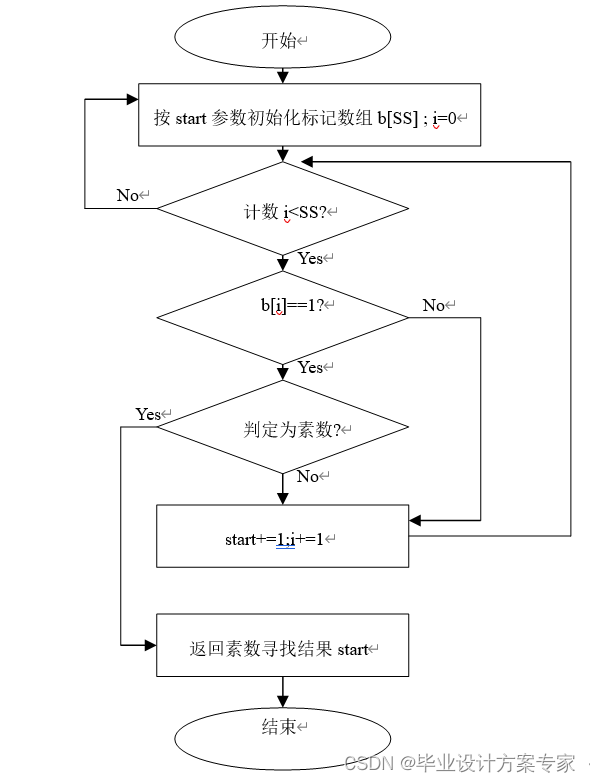4 二元一次不定方程

（1） 11 x - 49 y = 1 49 mod 11 = 5
（2） 11 x - 5 y = 1 11 mod 5 = 1
（3） x - 5 y = 1 5 mod 1 = 0

x=9;y=2即为所求。

5 RSA算法实现加密与解密

①生成密钥：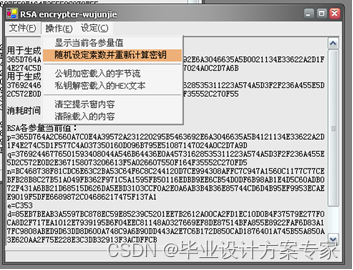public static int GetRandomString()//实现随机字串的获得
{
Random rnd = new Random();
Byte[]b=new Byte[System.Math.Max(RSAprimeplen1,RSAprimeplen2)];
s1="";
s2="";
for(int i=0;i<RSAprimeplen1;i++)
{
Byte tmp=System.Convert.ToByte(254.0*rnd.NextDouble());
if(tmp!=0)b[i]=tmp;else b[i]=1;			}
s1=wujunjie_rsa.FromASCIIByteArray(b);

for(int i=0;i<RSAprimeplen2;i++)
{
Byte tmp=System.Convert.ToByte(254.0*rnd.NextDouble());
if(tmp!=0)b[i]=tmp;else b[i]=1;
}
s2=wujunjie_rsa.FromASCIIByteArray(b);

return 1;}


②加密过程：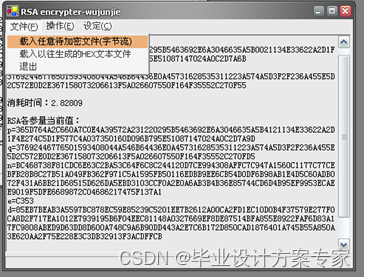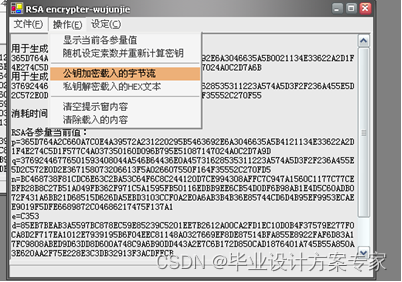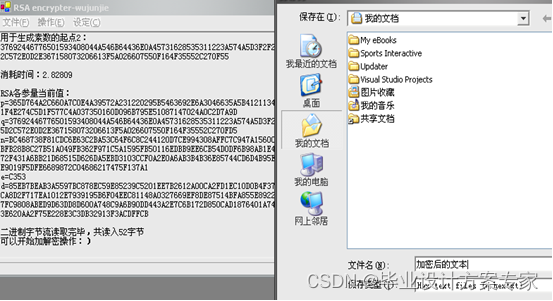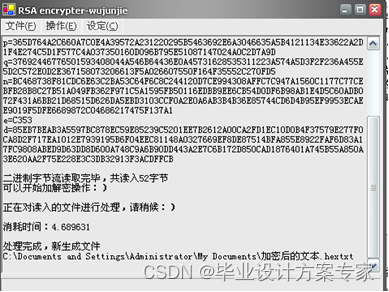private void menuItem10_Click(object sender, System.EventArgs e)//公钥加密
{
if(wujunjie_rsa.charlist.Count==0)
{
emptymsg em=new emptymsg(this);
em.Show();
return;
}

Stream myStream ;
SaveFileDialog saveFileDialog1 = new SaveFileDialog();

saveFileDialog1.Filter = "Hex text files (*.hextxt)|*.hextxt|All files (*.*)|*.*"  ;
saveFileDialog1.FilterIndex = 1 ;
saveFileDialog1.RestoreDirectory = true ;

if(saveFileDialog1.ShowDialog() == DialogResult.OK)
{
if((myStream=saveFileDialog1.OpenFile()) != null)
{
textBox1.Text+="\r\n正在对读入的文件进行处理，请稍候：）\r\n";
HighResolutionTimer timer = new HighResolutionTimer();
timer.Start();
using (StreamWriter sw = new StreamWriter(myStream))
{
sw.WriteLine("# RSA.HexText");
sw.WriteLine("#___________________________________________");

Byte []b=new Byte[wujunjie_rsa.RSAstep];
wujunjie_rsa.result_hexstrings.Clear();
progressBar1.Minimum=0;
progressBar1.Maximum=wujunjie_rsa.charlist.Count;
for(int i=0;i<wujunjie_rsa.charlist.Count;i+=System.Convert.ToInt32(wujunjie_rsa.RSAstep))
{
for(int j=0;j<wujunjie_rsa.RSAstep;j++)
{
b[j]=System.Convert.ToByte(wujunjie_rsa.charlist[i+j]);
}
string s;
wujunjie_rsa.RSA_san_en(b,wujunjie_rsa.RSAstep);
s=wujunjie_rsa.get_result_hexstring();
progressBar1.Value=i+1;
}

for(int i=0;i<wujunjie_rsa.result_hexstrings.Count;i++)
{
string hs=System.Convert.ToString(wujunjie_rsa.result_hexstrings[i]);
if(hs==null||hs=="")sw.WriteLine("0");
else sw.WriteLine(hs);
}

sw.WriteLine("#___________________________________________");
sw.Write("# ");
sw.WriteLine(DateTime.Now);

wujunjie_rsa.result_hexstrings.Clear();

}
myStream.Close();
timer.Stop();
textBox1.Text+="\r\n消耗时间："+timer.ElapsedTime+"\r\n";

textBox1.Text+="\r\n处理完成，新生成文件\r\n"+saveFileDialog1.FileName+"\r\n";
progressBar1.Value=0;
}
}
}


③解密过程：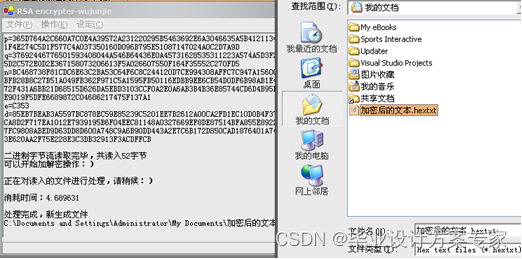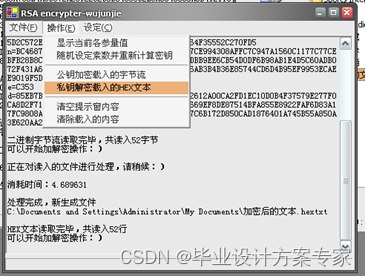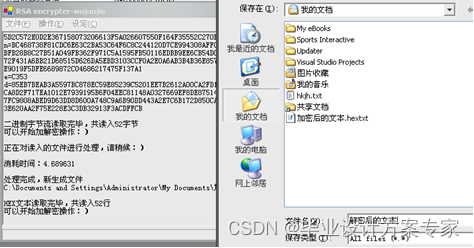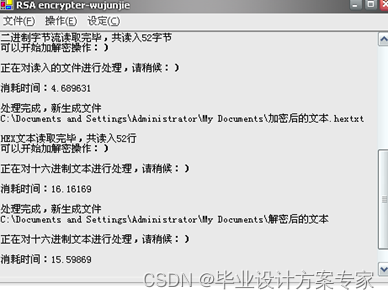private void menuItem8_Click(object sender, System.EventArgs e)//私钥解密
{
if(wujunjie_rsa.hextxtlist.Count==0)
{
emptymsg em=new emptymsg(this);
em.Show();
return;
}

Stream myStream ;
SaveFileDialog saveFileDialog1 = new SaveFileDialog();

saveFileDialog1.Filter = "Text files (*.txt)|*.txt|All files (*.*)|*.*"  ;
saveFileDialog1.FilterIndex = 2 ;
saveFileDialog1.RestoreDirectory = true ;

if(saveFileDialog1.ShowDialog() == DialogResult.OK)
{
if((myStream=saveFileDialog1.OpenFile()) != null)
{
textBox1.Text+="\r\n正在对十六进制文本进行处理，请稍候：）\r\n";
HighResolutionTimer timer = new HighResolutionTimer();
timer.Start();
using (BinaryWriter bn = new BinaryWriter(myStream))
{
progressBar1.Minimum=0;
progressBar1.Maximum=wujunjie_rsa.hextxtlist.Count;
for(int i=0;i<wujunjie_rsa.hextxtlist.Count;i++)
{
wujunjie_rsa.RSA_san_dn_hexstring(System.Convert.ToString(wujunjie_rsa.hextxtlist[i]));

for(uint j=0;j<wujunjie_rsa.RSAstep;j++)
{
bn.Write(wujunjie_rsa.get_result_byte(j));
}
progressBar1.Value=i+1;

}
}

myStream.Close();
timer.Stop();
textBox1.Text+="\r\n消耗时间："+timer.ElapsedTime+"\r\n";

textBox1.Text+="\r\n处理完成，新生成文件\r\n"+saveFileDialog1.FileName+"\r\n";
progressBar1.Value=0;}
}
}


6 核心类库综述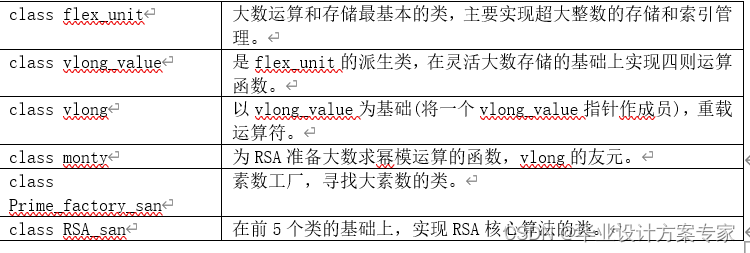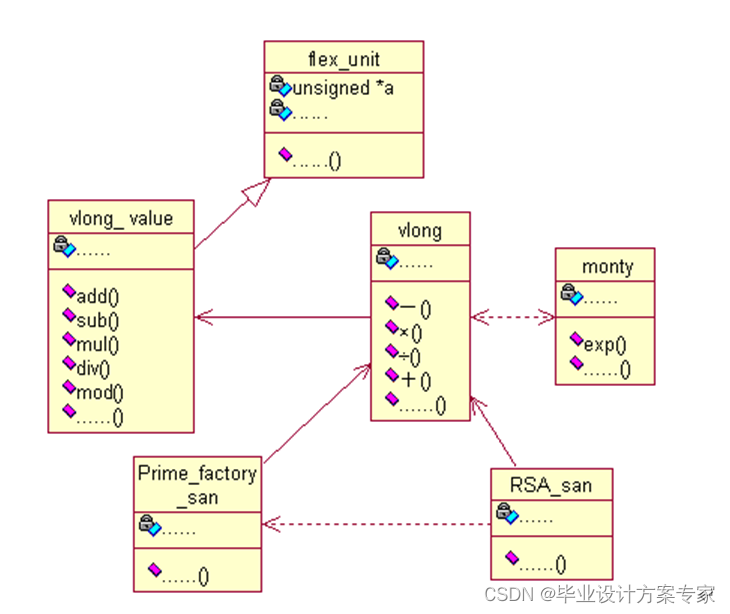2.2.2 封装C++核心类库的DLL组件

2.2.3 引用DLL的.Net类与实现文件操作功能的窗体应用程序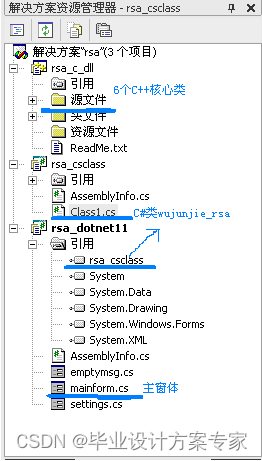3 软件整体测试与分析改进
3.1 编写测试各项性能需要的精确计时类

3.2 测试数据与分析改进
3.2.1 密钥生成测试

1 测试加密使用的n的位数对耗时的影响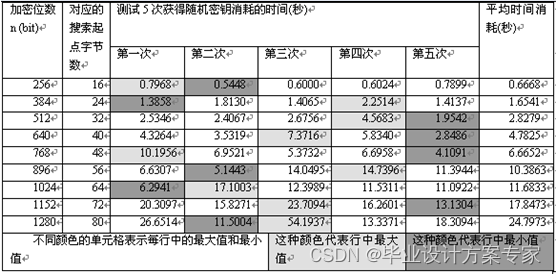3.2.2 数据输入输出测试

3.2.3 加密解密测试

1 用同样的密钥对不同大小的文件公钥加密、私钥解密，各自消耗的时间与待加密文件大小的关系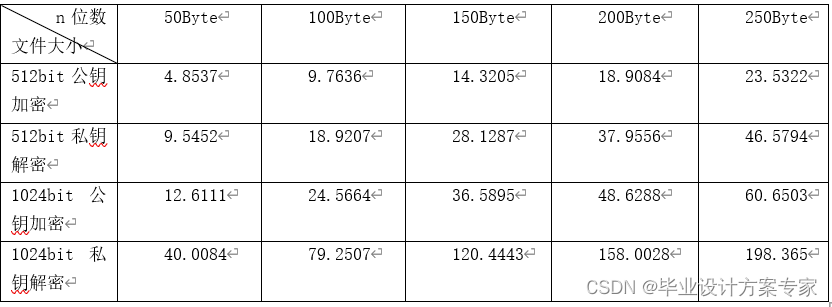3 在更快的PC，对进行文件加密测试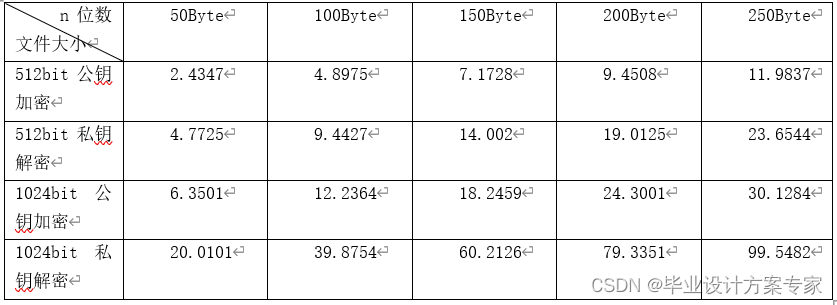① 软件消耗时间的运算，大部分集中在C++核心类库，即RSA相关的各种运算。其中，幂模运算和寻找素数对时间的消耗最大，在核心优化时应优先考虑。
② 文件输入输出消耗时间其次，因为磁盘读写速度要远远低于内存读写速度。所以，应该将频繁的读写操作尽量集中到内存，然后一次性写入磁盘。

① 在要对文件进行加密解密的时候，先将文件按一定的数据结构读入内存，然后进行加密或解密操作。运算数据都读取自内存。
② 在对加密或解密完成的数据进行写出的时候，都是将其直接写到指定好的文件，即直接写入磁盘。这是因为，考虑到中途可能因为意外断电等原因引起操作中断，为了保护已经花费时间运算完成的数据，将其直接写入磁盘。
③ 在关键算法上做进一步优化，例如在寻找素数时，素数测试使用更快速的算法。
④ 对C++核心类库进行重点优化，使其运算效率尽可能提高。其中包括对各类之间的组织细节、各程序模块的具体编写等，进行全面细致的检查和修改，例如将大数据类型以对象指针传递而不拷贝，将简单的for循环展开等。

 华罗庚.数论导引[M].北京：科学出版社.1979.6。
 蔡乐才,张仕斌.应用密码学[M].北京：中国电力出版社.2005.2。
 沈世镒,陈鲁生.现代密码学[M].北京：科学出版社.2004.1。
 施向东,董平.基于RSA算法的一种新的加密核设计[J].北京：微计算机信息,2005,12: 39-41。
 吴春明,秦建. RSA在软件实现中的算法研究[J].北京：农业网络信息,2006, 08:41-46。
范益波,曾晓洋.基于16位的Montgomery模乘法器设计[J].北京：通讯学报 2006,27(4):107-113。
 陈发来,李尚志.数学实验——素数[M].北京：高等教育出版社.2004.8。

## 5、资源下载

03-121065
02-24304
03-121849
04-30399
01-08370
11-08104
04-144011
06-033583
02-262929
04-076718
08-13233
03-05969
09-23120
09-25174

### “相关推荐”对你有帮助么？

•非常没帮助
•没帮助
•一般
•有帮助
•非常有帮助

©️2022 CSDN 皮肤主题：深蓝海洋 设计师：CSDN官方博客被折叠的  条评论 为什么被折叠?到【灌水乐园】发言¥2 ¥4 ¥6 ¥10 ¥20余额支付 (余额：-- )扫码支付获取中扫码支付点击重新获取扫码支付1.余额是钱包充值的虚拟货币，按照1:1的比例进行支付金额的抵扣。
2.余额无法直接购买下载，可以购买VIP、C币套餐、付费专栏及课程。余额充值